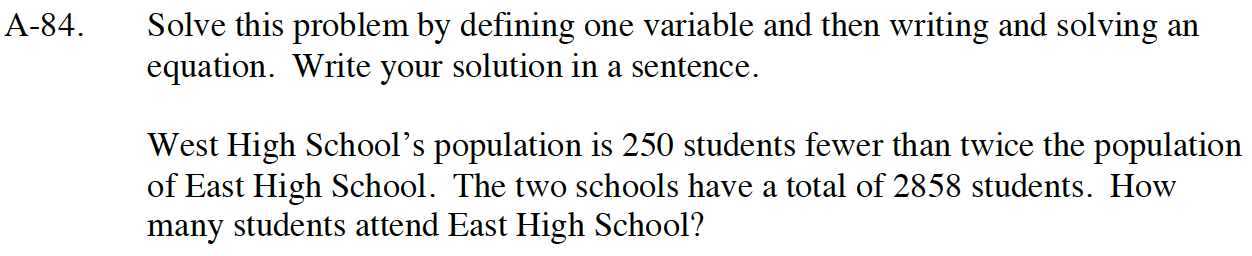Home > CC4 > Chapter A > Lesson A.1.8 > ProblemA-84

A-84.

Solve this problem by defining one variable and then writing and solving an equation. Write your solution in a sentence.

West High School’s population is 250 students fewer than twice the population of East High School. The two schools have a total of 2858 students. How many students attend East High School? Homework Help ✎Define a variable.

x = the number of East High School students

Write an expression that defines the number of West High students in terms of the variable.

2x − 250 = the number of West High students

Write an equation using the total population of the two schools.

x + (2x − 250) = 2858

Solve for x.